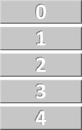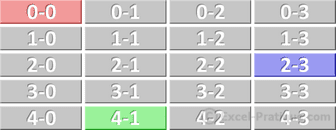# VBA Course: Variables (continued)

## Arrays

While variables only allow us to store one value each, arrays make it possible to store many values and they work almost exactly the same way.

Here are some examples of declarations:

`'Sample variable declarationDim var1 As String    'Sample 1 dimensional array declarationDim array1(4) As String    'Sample 2 dimensional array declarationDim array2(4, 3) As String    'Sample 3 dimensional array declarationDim array3(4, 3, 2) As String`

The 1 dimensional array:

`'Sample 1 dimensional array declarationDim array1(4) As String`

There is only one number in parentheses in this declaration, which means that it is a one dimensional array. This number sets the size of the array. array1(4) is an array in which the cells are numbered from 0 to 4, which means that it is an array with 5 cells:

`'Assigning values to the 5 cellsarray1(0) = "Value of cell 0"array1(1) = "Value of cell 1"array1(2) = "Value of cell 2"array1(3) = "Value of cell 3"array1(4) = "Value of cell 4"`Important: the first cell in an array is numbered 0.

Another example, a two dimensional array:

`'Sample declaration of a 2 dimensional arrayDim array2(4, 3) As String``'Assigning values to three colored cellsarray2(0, 0) = "Red cell value"array2(4, 1) = "Green cell value"array2(2, 3) = "Blue cell value"`

## Constants

Like variables, constants can be used to store values, but the difference is that they can't be modified (thus their name).

For example, we could add a constant to avoid having to repeat a number like 6.87236476641:

`Sub const_example()    Cells(1, 1) = Cells(1, 2) * 6.87236476641    Cells(2, 1) = Cells(2, 2) * 6.87236476641    Cells(3, 1) = Cells(3, 2) * 6.87236476641    Cells(4, 1) = Cells(4, 2) * 6.87236476641    Cells(5, 1) = Cells(5, 2) * 6.87236476641End Sub`

This makes the code much easier to read (important parts in particular) and makes it much easier to change the value of the constant, should you need to:

`Sub const_example()   'Declaration of a constant + assignment of value    Const ANNUAL_RATE As Double = 6.87236476641        Cells(1, 1) = Cells(1, 2) * ANNUAL_RATE    Cells(2, 1) = Cells(2, 2) * ANNUAL_RATE    Cells(3, 1) = Cells(3, 2) * ANNUAL_RATE    Cells(4, 1) = Cells(4, 2) * ANNUAL_RATE    Cells(5, 1) = Cells(5, 2) * ANNUAL_RATEEnd Sub`

## The scope of variables

If a variable is declared at the beginning of a procedure (Sub), it can only be used within this same procedure. The value of the variable will not be maintained after the execution of the procedure.

`Sub procedure1()   Dim var1 As Integer   ' => Use of a variable only within a procedureEnd SubSub procedure2()   ' => var1 cannot be used hereEnd Sub`

In order to use a variable in any of the procedures within a module, all you have to do is declare it at the beginning of the module. And if you declare a variable this way, its value will be maintained until the workbook is closed.

`Dim var1 As IntegerSub procedure1()   ' => var1 can be used hereEnd SubSub procedure2()   ' => var1 can also be used hereEnd Sub`

If you want to be able to use a variable in any module, on the same principle as the previous example, all you have to do is replace Dim with Global:

`Global var1 As Integer`

To maintain the value of a variable after the execution of the procedure in which it appears, replace Dim with Static:

`Sub procedure1()    Static var1 As IntegerEnd Sub`

To maintain the values of all the variables in a procedure, add Static before Sub:

`Static Sub procedure1()    Dim var1 As IntegerEnd Sub`

## Create your own type of variable

We won't spend very much time on this point. Here is an example:

`'Creation of a variable typeType guests    last_name As String    first_name As StringEnd Type    Sub variables()    'Declaration    Dim p1 As guests        'Assigning values to p1    p1.last_name = "Smith"    p1.first_name = "John"        'Example of use    MsgBox p1.last_name & " " & p1.first_nameEnd Sub`